Anzeige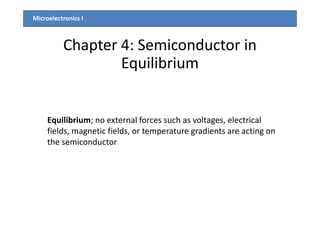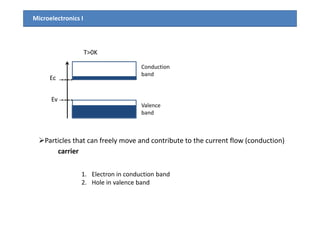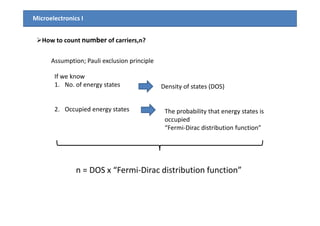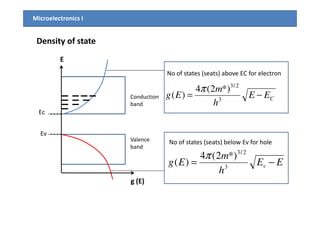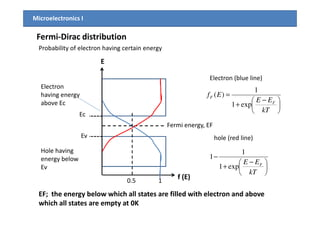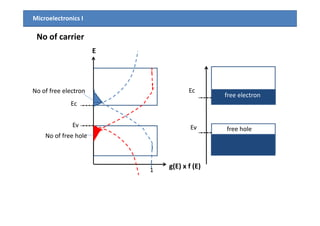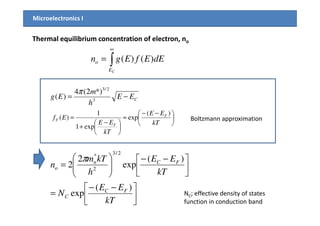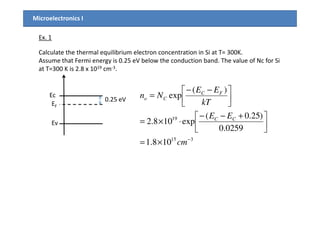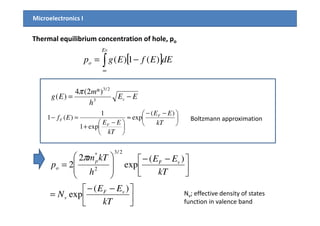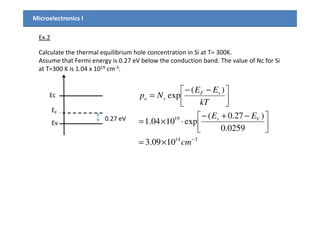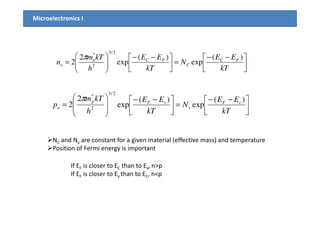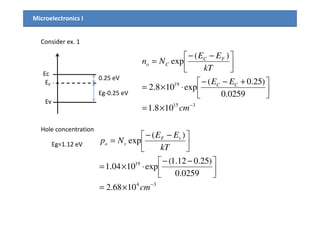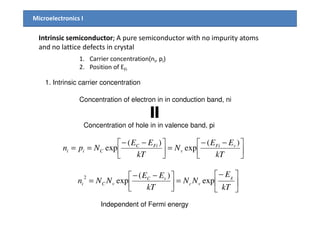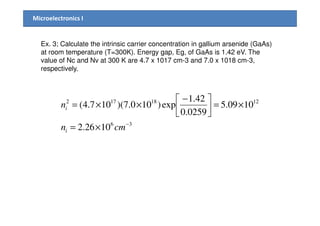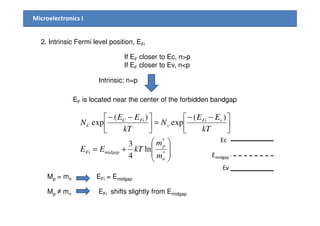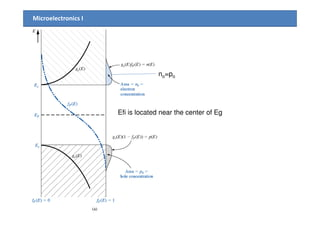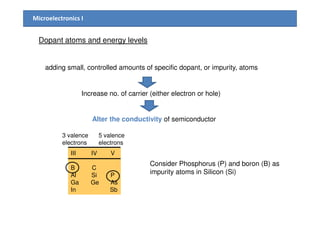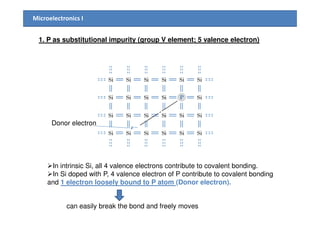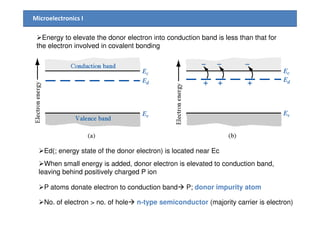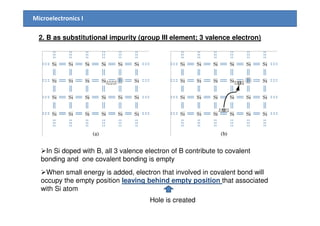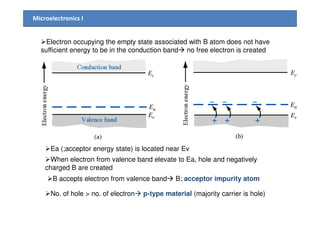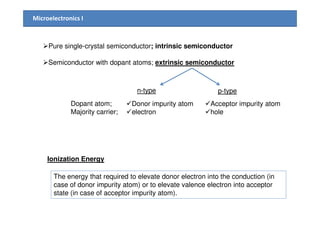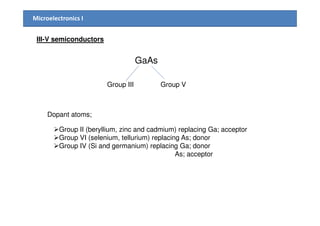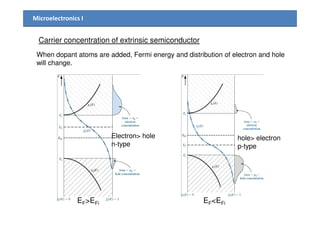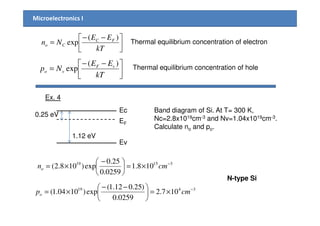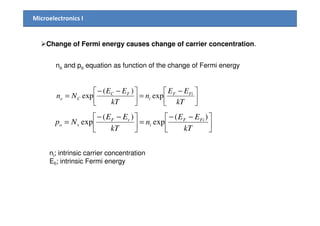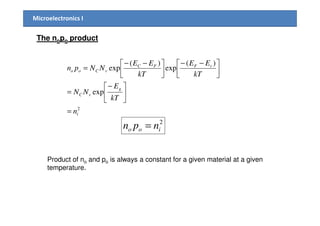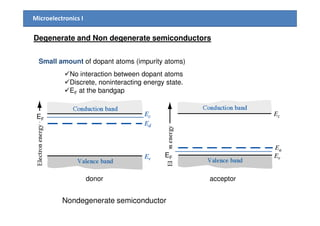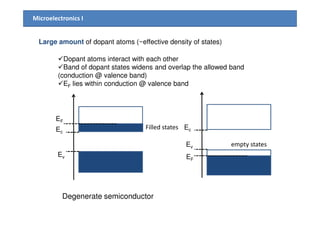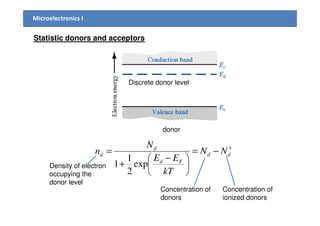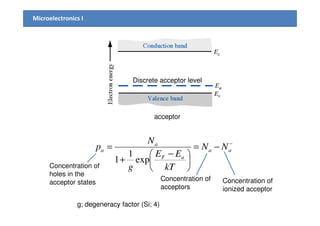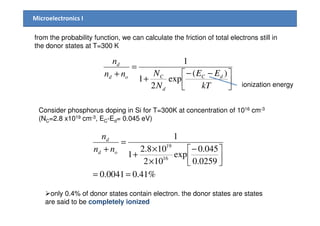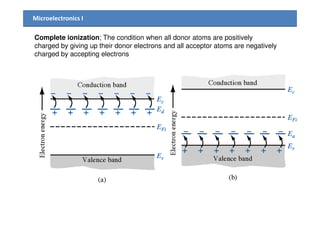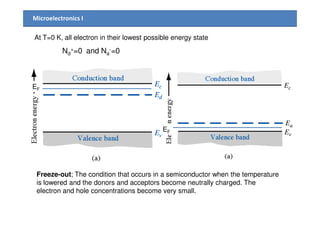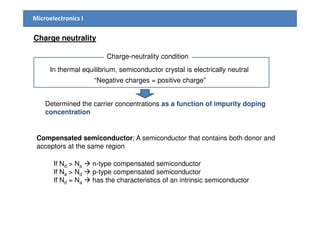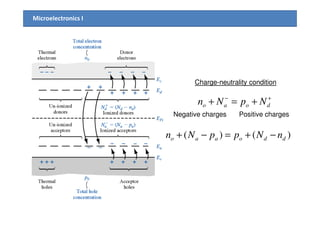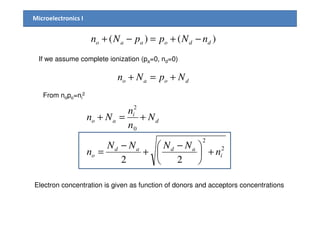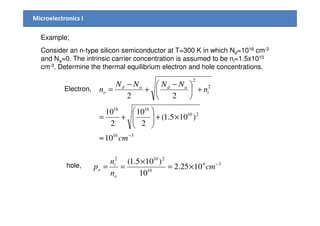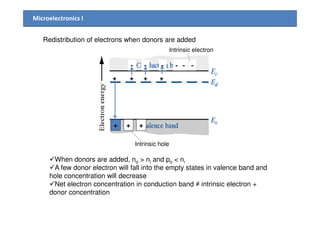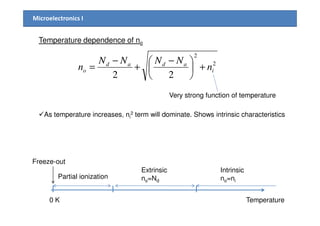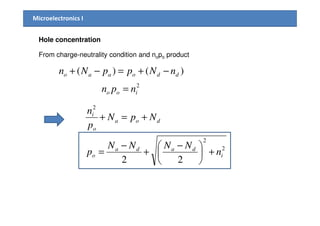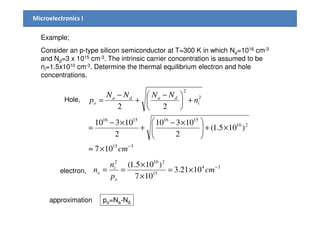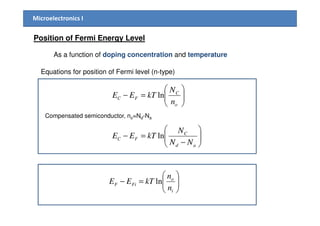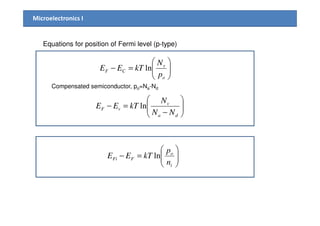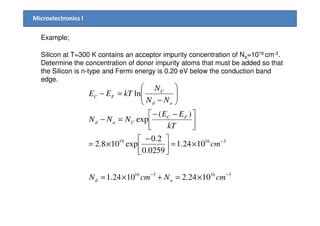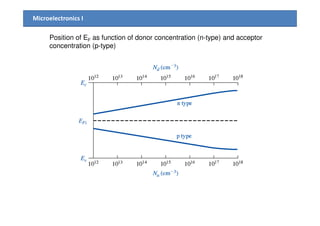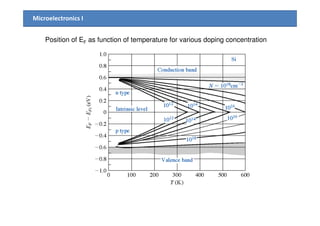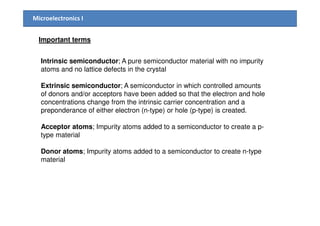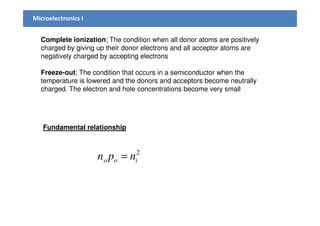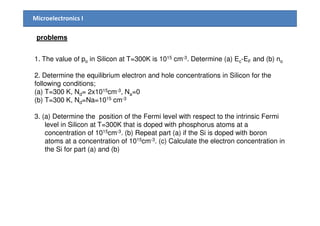1 von 50
Anzeige

### Chapter4 semiconductor in equilibrium

1. Microelectronics I Chapter 4: Semiconductor in Equilibrium Equilibrium; no external forces such as voltages, electrical fields, magnetic fields, or temperature gradients are acting on the semiconductor
2. Microelectronics I e e Ec Ev Conduction band Valence band T>0K e band Particles that can freely move and contribute to the current flow (conduction) 1. Electron in conduction band 2. Hole in valence band carrier
3. Microelectronics I How to count number of carriers,n? If we know 1. No. of energy states 2. Occupied energy states Density of states (DOS) The probability that energy states is Assumption; Pauli exclusion principle 2. Occupied energy states The probability that energy states is occupied “Fermi-Dirac distribution function” n = DOS x “Fermi-Dirac distribution function”
4. e Ec Conduction band CEE h m Eg −= 3 2/3 *)2(4 )( π No of states (seats) above EC for electron Microelectronics I Density of state E e Ec Ev Valence band EE h m Eg v −= 3 2/3 *)2(4 )( π No of states (seats) below Ev for hole g (E)
5. Fermi-Dirac distribution Microelectronics I e Ec Probability of electron having certain energy E Electron (blue line)       − + = kT EE Ef F F exp1 1 )( Electron having energy above Ec e Ec Ev f (E)10.5 Fermi energy, EF EF; the energy below which all states are filled with electron and above which all states are empty at 0K  kT hole (red line)       − + − kT EE F exp1 1 1 Hole having energy below Ev
6. e Ec E Microelectronics I No of carrier No of free electron e Ec free electron e Ev g(E) x f (E)1 No of free hole e Ev free hole
7. Microelectronics I Thermal equilibrium concentration of electron, no ∫ ∞ = CE o dEEfEgn )()( CEE h m Eg −= 3 2/3 *)2(4 )( π  −− ≈= EE )(1       −− ≈       − + = kT EE kT EE Ef F F F )( exp exp1 1 )( Boltzmann approximation       −− =       −−       = kT EE N kT EE h kTm n FC C FCn o )( exp )( exp 2 2 2/3 2 * π NC; effective density of states function in conduction band
8. Microelectronics I Ex. 1 Calculate the thermal equilibrium electron concentration in Si at T= 300K. Assume that Fermi energy is 0.25 eV below the conduction band. The value of Nc for Si at T=300 K is 2.8 x 1019 cm-3. Ec EF 0.25 eV )25.0( )( exp  +−−       −− = EE kT EE Nn FC Co Ev 315 19 108.1 0259.0 )25.0( exp108.2 − ×=       +−− ⋅×= cm EE CC
9. Thermal equilibrium concentration of hole, po Microelectronics I [ ]∫∞ −= Ev o dEEfEgp )(1)( EE h m Eg v −= 3 2/3 *)2(4 )( π  −− ≈=− EE )(1       −− ≈       − + =− kT EE kT EE Ef F F F )( exp exp1 1 )(1 Boltzmann approximation       −− =       −−         = kT EE N kT EE h kTm p vF v vFp o )( exp )( exp 2 2 2/3 2 * π Nv; effective density of states function in valence band
10. Microelectronics I Ex.2 Calculate the thermal equilibrium hole concentration in Si at T= 300K. Assume that Fermi energy is 0.27 eV below the conduction band. The value of Nc for Si at T=300 K is 1.04 x 1019 cm-3. Ec EF )27.0( )( exp  −+−       −− = EE kT EE Np vF vo Ev EF 0.27 eV 314 19 1009.3 0259.0 )27.0( exp1004.1 − ×=       −+− ⋅×= cm EE Vv
11. Microelectronics I       −− =      −−       = kT EE N kT EE h kTm n FC C FCn o )( exp )( exp 2 2 2/3 2 * π       −− =      −−         = kT EE N kT EE h kTm p vF v vFp o )( exp )( exp 2 2 2/3 2 * π  kTkTh NC and Nv are constant for a given material (effective mass) and temperature Position of Fermi energy is important If EF is closer to EC than to Ev, n>p If EF is closer to Ev than to EC, n<p
12. Microelectronics I Consider ex. 1 Ec Ev EF 0.25 eV 315 19 108.1 0259.0 )25.0( exp108.2 )( exp − ×=       +−− ⋅×=       −− = cm EE kT EE Nn CC FC Co Eg-0.25 eV Hole concentration Eg=1.12 eV 34 19 1068.2 0259.0 )25.012.1( exp1004.1 )( exp − ×=     −− ⋅×=       −− = cm kT EE Np vF vo
13. Microelectronics I Intrinsic semiconductor; A pure semiconductor with no impurity atoms and no lattice defects in crystal 1. Carrier concentration(ni, pi) 2. Position of EFi 1. Intrinsic carrier concentration Concentration of electron in in conduction band, ni Concentration of hole in in valence band, pi       −− =      −− == kT EE N kT EE Npn vFi v FiC Cii )( exp )( exp      − =      −− = kT E NN kT EE NNn g vc vC vCi exp )( exp 2 Independent of Fermi energy
14. Microelectronics I Ex. 3; Calculate the intrinsic carrier concentration in gallium arsenide (GaAs) at room temperature (T=300K). Energy gap, Eg, of GaAs is 1.42 eV. The value of Nc and Nv at 300 K are 4.7 x 1017 cm-3 and 7.0 x 1018 cm-3, respectively. 1218172 1009.5 0259.0 42.1 exp)100.7)(107.4( ×=    − ××=ni 36 1026.2 0259.0 − ×=  cmni
15. Microelectronics I 2. Intrinsic Fermi level position, EFi If EF closer to Ec, n>p If EF closer to Ev, n<p Intrinsic; n=p EF is located near the center of the forbidden bandgap  −− −− )()( EEEE         +=       −− =      −− * * ln 4 3 )( exp )( exp n p midgapFi vFi v FiC C m m kTEE kT EE N kT EE N Ec Ev Emidgap Mp ≠ mn Mp = mn EFi = Emidgap EFi shifts slightly from Emidgap
16. Microelectronics I Efi is located near the center of Eg no=po Efi is located near the center of Eg
17. Microelectronics I Dopant atoms and energy levels adding small, controlled amounts of specific dopant, or impurity, atoms Increase no. of carrier (either electron or hole) Alter the conductivity of semiconductor III IV V B C Al Si P Ga Ge As In Sb 3 valence electrons 5 valence electrons Consider Phosphorus (P) and boron (B) as impurity atoms in Silicon (Si)
18. Microelectronics I 1. P as substitutional impurity (group V element; 5 valence electron) In intrinsic Si, all 4 valence electrons contribute to covalent bonding. In Si doped with P, 4 valence electron of P contribute to covalent bonding and 1 electron loosely bound to P atom (Donor electron). Donor electron can easily break the bond and freely moves
19. Microelectronics I Energy to elevate the donor electron into conduction band is less than that for the electron involved in covalent bonding Ed(; energy state of the donor electron) is located near Ec When small energy is added, donor electron is elevated to conduction band, leaving behind positively charged P ion P atoms donate electron to conduction band P; donor impurity atom No. of electron > no. of hole n-type semiconductor (majority carrier is electron)
20. Microelectronics I 2. B as substitutional impurity (group III element; 3 valence electron) In Si doped with B, all 3 valence electron of B contribute to covalent bonding and one covalent bonding is empty When small energy is added, electron that involved in covalent bond will occupy the empty position leaving behind empty position that associated with Si atom Hole is created
21. Microelectronics I Electron occupying the empty state associated with B atom does not have sufficient energy to be in the conduction band no free electron is created Ea (;acceptor energy state) is located near Ev When electron from valence band elevate to Ea, hole and negatively charged B are created B accepts electron from valence band B; acceptor impurity atom No. of hole > no. of electron p-type material (majority carrier is hole)
22. Microelectronics I Pure single-crystal semiconductor; intrinsic semiconductor Semiconductor with dopant atoms; extrinsic semiconductor p-typen-type Dopant atom; Majority carrier; Donor impurity atom electron Acceptor impurity atom holeMajority carrier; electron hole Ionization Energy The energy that required to elevate donor electron into the conduction (in case of donor impurity atom) or to elevate valence electron into acceptor state (in case of acceptor impurity atom).
23. Microelectronics I III-V semiconductors GaAs Group III Group V Dopant atoms;Dopant atoms; Group II (beryllium, zinc and cadmium) replacing Ga; acceptor Group VI (selenium, tellurium) replacing As; donor Group IV (Si and germanium) replacing Ga; donor As; acceptor
24. Microelectronics I Carrier concentration of extrinsic semiconductor When dopant atoms are added, Fermi energy and distribution of electron and hole will change. EF>EFi EF<EFi Electron> hole n-type hole> electron p-type
25. Microelectronics I       −− = kT EE Nn FC Co )( exp       −− = kT EE Np vF vo )( exp Thermal equilibrium concentration of electron Thermal equilibrium concentration of hole Ex. 4 Ec 0.25 eV Band diagram of Si. At T= 300 K,Ec Ev EF 1.12 eV 0.25 eV Band diagram of Si. At T= 300 K, Nc=2.8x1019cm-3 and Nv=1.04x1019cm-3. Calculate no and po. 31519 108.1 0259.0 25.0 exp)108.2( − ×=      − ×= cmno 3419 107.2 0259.0 )25.012.1( exp)1004.1( − ×=      −− ×= cmpo N-type Si
26. Microelectronics I Change of Fermi energy causes change of carrier concentration. no and po equation as function of the change of Fermi energy       − =      −− = kT EE n kT EE Nn FiF i FC Co exp )( exp  −− −− EEEE )()(       −− =      −− = kT EE n kT EE Np FiF i vF vo )( exp )( exp ni; intrinsic carrier concentration Efi; intrinsic Fermi energy
27. Microelectronics I The nopo product 2 exp )( exp )( exp i g vC vFFC vCoo n kT E NN kT EE kT EE NNpn =      − =       −−       −− = in= 2 ioo npn = Product of no and po is always a constant for a given material at a given temperature.
28. Microelectronics I Degenerate and Non degenerate semiconductors Small amount of dopant atoms (impurity atoms) No interaction between dopant atoms Discrete, noninteracting energy state. EF at the bandgap E donor acceptor Nondegenerate semiconductor EF EF
29. Large amount of dopant atoms (~effective density of states) Microelectronics I Dopant atoms interact with each other Band of dopant states widens and overlap the allowed band (conduction @ valence band) EF lies within conduction @ valence band e e e Ec Ev Filled states EF e Ec Ev empty states EF Degenerate semiconductor
30. Microelectronics I Statistic donors and acceptors Discrete donor level donor + −=       − + = dd Fd d d NN kT EE N n exp 2 1 1Density of electron occupying the donor level Concentration of donors Concentration of ionized donors
31. Microelectronics I acceptor Discrete acceptor level acceptor − −=       − + = aa aF a a NN kT EE g N p exp 1 1 Concentration of holes in the acceptor states Concentration of acceptors Concentration of ionized acceptor g; degeneracy factor (Si; 4)
32. Microelectronics I from the probability function, we can calculate the friction of total electrons still in the donor states at T=300 K     −− + = + kT EE N Nnn n dC d Cod d )( exp 2 1 1 Consider phosphorus doping in Si for T=300K at concentration of 1016 cm-3 ionization energy Consider phosphorus doping in Si for T=300K at concentration of 10 cm (NC=2.8 x1019 cm-3, EC-Ed= 0.045 eV) %41.00041.0 0259.0 045.0 exp 102 108.2 1 1 16 19 ==    − × × + = + od d nn n only 0.4% of donor states contain electron. the donor states are states are said to be completely ionized
33. Microelectronics I Complete ionization; The condition when all donor atoms are positively charged by giving up their donor electrons and all acceptor atoms are negatively charged by accepting electrons
34. Microelectronics I At T=0 K, all electron in their lowest possible energy state Nd +=0 and Na -=0 EF EF Freeze-out; The condition that occurs in a semiconductor when the temperature is lowered and the donors and acceptors become neutrally charged. The electron and hole concentrations become very small.
35. Microelectronics I Charge neutrality In thermal equilibrium, semiconductor crystal is electrically neutral “Negative charges = positive charge” Determined the carrier concentrations as a function of impurity doping concentration Charge-neutrality condition Compensated semiconductor; A semiconductor that contains both donor and acceptors at the same region If Nd > Na n-type compensated semiconductor If Na > Nd p-type compensated semiconductor If Nd = Na has the characteristics of an intrinsic semiconductor concentration
36. Microelectronics I Charge-neutrality condition +− +=+ doao NpNn Negative charges Positive chargesNegative charges Positive charges )()( ddoaao nNppNn −+=−+
37. Microelectronics I )()( ddoaao nNppNn −+=−+ If we assume complete ionization (pa=0, nd=0) doao NpNn +=+ From nopo=ni 2 2 i N n Nn +=+ 2 2 0 22 i adad o d i ao n NNNN n N n n Nn +      − + − = +=+ Electron concentration is given as function of donors and acceptors concentrations
38. Microelectronics I Example; Consider an n-type silicon semiconductor at T=300 K in which Nd=1016 cm-3 and Na=0. The intrinsic carrier concentration is assumed to be ni=1.5x1010 cm-3. Determine the thermal equilibrium electron and hole concentrations. 210 1616 2 2 )105.1( 1010 22 ×+    += +      − + − = n NNNN n i adad o Electron, 316 210 10 )105.1( 2 10 2 10 − ≈ ×+      += cm hole, 34 16 2102 1025.2 10 )105.1( − ×= × == cm n n p o i o
39. Microelectronics I Redistribution of electrons when donors are added + + + + - - - - - - Intrinsic electron + + + Intrinsic hole When donors are added, no > ni and po < ni A few donor electron will fall into the empty states in valence band and hole concentration will decrease Net electron concentration in conduction band ≠ intrinsic electron + donor concentration
40. Microelectronics I Temperature dependence of no 2 2 22 i adad o n NNNN n +      − + − = Very strong function of temperature As temperature increases, n2 term will dominate. Shows intrinsic characteristicsAs temperature increases, ni 2 term will dominate. Shows intrinsic characteristics 0 K Temperature Freeze-out Partial ionization Extrinsic no=Nd Intrinsic no=ni
41. Microelectronics I Hole concentration From charge-neutrality condition and nopo product )()( ddoaao nNppNn −+=−+ 2 ioo npn = 2 i NpN n +=+ 2 2 22 i dada o doa o i n NNNN p NpN p n +      − + − = +=+
42. Microelectronics I Example; Consider an p-type silicon semiconductor at T=300 K in which Na=1016 cm-3 and Nd=3 x 1015 cm-3. The intrinsic carrier concentration is assumed to be ni=1.5x1010 cm-3. Determine the thermal equilibrium electron and hole concentrations. 2 2 22 +      − + − = n NNNN p i dada o Hole, 315 210 15161516 107 )105.1( 2 10310 2 10310 − ×≈ ×+      ×− + ×− = cm electron, 34 15 2102 1021.3 107 )105.1( − ×= × × == cm p n n o i o po=Na-Ndapproximation
43. Microelectronics I Position of Fermi Energy Level As a function of doping concentration and temperature Equations for position of Fermi level (n-type)       =− o C FC n N kTEE ln Compensated semiconductor, n =N -N       − =− ad C FC NN N kTEE ln Compensated semiconductor, no=Nd-Na       =− i o FiF n n kTEE ln
44. Microelectronics I Equations for position of Fermi level (p-type)       =− o v CF p N kTEE ln Compensated semiconductor, po=Na-Nd       − =− da v vF NN N kTEE ln     − da NN       =− i o FFi n p kTEE ln
45. Microelectronics I Example; Silicon at T=300 K contains an acceptor impurity concentration of Na=1016 cm-3. Determine the concentration of donor impurity atoms that must be added so that the Silicon is n-type and Fermi energy is 0.20 eV below the conduction band edge. )( ln  −−       − =− EE NN N kTEE ad C FC 31619 1024.1 0259.0 2.0 exp108.2 )( exp − ×=    − ×=       −− =− cm kT EE NNN FC Cad 316316 1024.21024.1 −− ×=+×= cmNcmN ad
46. Microelectronics I Position of EF as function of donor concentration (n-type) and acceptor concentration (p-type)
47. Microelectronics I Position of EF as function of temperature for various doping concentration
48. Microelectronics I Important terms Intrinsic semiconductor; A pure semiconductor material with no impurity atoms and no lattice defects in the crystal Extrinsic semiconductor; A semiconductor in which controlled amounts of donors and/or acceptors have been added so that the electron and hole concentrations change from the intrinsic carrier concentration and a preponderance of either electron (n-type) or hole (p-type) is created.preponderance of either electron (n-type) or hole (p-type) is created. Acceptor atoms; Impurity atoms added to a semiconductor to create a p- type material Donor atoms; Impurity atoms added to a semiconductor to create n-type material
49. Microelectronics I Complete ionization; The condition when all donor atoms are positively charged by giving up their donor electrons and all acceptor atoms are negatively charged by accepting electrons Freeze-out; The condition that occurs in a semiconductor when the temperature is lowered and the donors and acceptors become neutrally charged. The electron and hole concentrations become very small Fundamental relationship 2 ioo npn =
50. Microelectronics I problems 1. The value of po in Silicon at T=300K is 1015 cm-3. Determine (a) Ec-EF and (b) no 2. Determine the equilibrium electron and hole concentrations in Silicon for the following conditions; (a) T=300 K, Nd= 2x1015cm-3, Na=0 (b) T=300 K, Nd=Na=1015 cm-3 3. (a) Determine the position of the Fermi level with respect to the intrinsic Fermi3. (a) Determine the position of the Fermi level with respect to the intrinsic Fermi level in Silicon at T=300K that is doped with phosphorus atoms at a concentration of 1015cm-3. (b) Repeat part (a) if the Si is doped with boron atoms at a concentration of 1015cm-3. (c) Calculate the electron concentration in the Si for part (a) and (b)
Anzeige